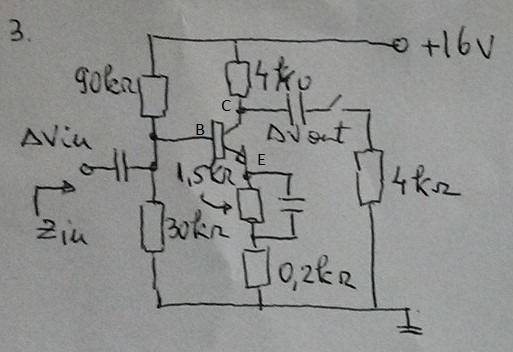# Calculating the amplification of a transistor

## Homework Statement

In the circuit shown below, I have to find the amplification for when the commutator which connects to the 4 kΩ resistance is open, respectively closed. We know the Beta factor to be 200, and the current through the base is negligible. The transistor is made of silicone. (Junctions conduct at 0.6 V)## Homework Equations

Av = Re/(Re+re), Av = amplification, Re = external resistance of the emitter, re = internal resistance of the emitter
re = Vt/Ic, Vt = 25 mV (constant for given temperature), Ic is one of the reasons I am asking this question.

## The Attempt at a Solution

For when it's open, I only took into account the 0.2 kΩ resistance in the emitter as the current flows through the capacitor integrally. I also found the current through the collector to be approximately 2 mA, with which I calculated re and thus the amplification. Now, for when the commutator is closed, I've been told by my professor that the current is maintained on the collector part, therefore I calculated the voltage in C. Having earlier obtained a value for the voltage in B of 4V, I simply added 0.6 V. Now, knowing the voltage in C, and that it's connected to a point of 0 potential through the branch with the commutator, I applied Ohm's law to find the current through the load (the 4 kΩ resistance on said branch). Further, I applied Kirchoff 1 to find the current that actually goes to the transistor from the collector, which I found to be about 0.85 mA. I recalculated re using that value for Ic (0.85 mA). Re remained the same.
I want to know if my method is correct, and a bit of a hint on how to get it right if it's not. (Which is rather likely, I am a Physics major so this subject makes less sense to me than most.)

## Answers and Replies

NascentOxygen
Staff Emeritus
Science Advisor
therefore I calculated the voltage in C. Having earlier obtained a value for the voltage in B of 4V, I simply added 0.6 V.
This is not correct for voltage at C. If you need to find it, the DC voltage at C is found as Vcc – IcRc.

It is not satisfactory to write a narrative about your calculations, we need to see them. Please attach a neat copy of your calculations (or compose it using Latex).

•Keiner Nichts
Oh, thank you, with that, I have managed to correctly determine the amplification without the load, as being -Rc/(Re+re). Now, Rc seems to be tied in parallel with the load because they have the same voltage difference on them. I am wondering, if Rc's resistance would not be equal to the load's, would them being tied in parallel still hold true?

NascentOxygen
Staff Emeritus
Science Advisor
When considering midband AC, the load is parallel to RC regardless of the ohmic values.

•Keiner Nichts
Thanks a bunch!

NascentOxygen
Staff Emeritus
Science Advisor
When considering midband AC, the load is parallel to RC regardless of the ohmic values.
Because current from the collector sees 2 independent paths to ground: (i) through the low impedance coupling capacitor and then RL to ground, or (ii) through RC and then through the low AC impedance to ground inside the low internal resistance of the ideal voltage source.

•Keiner Nichts